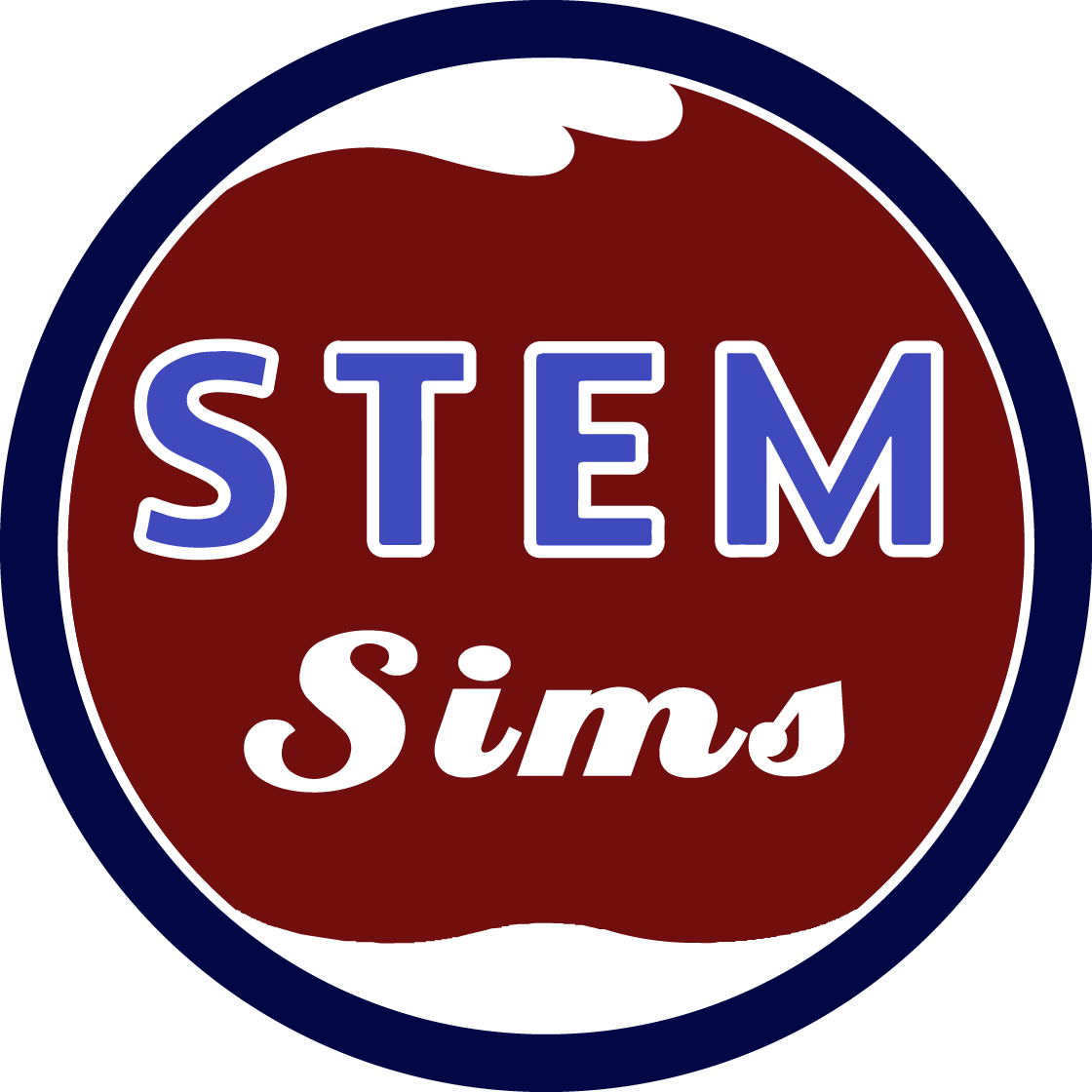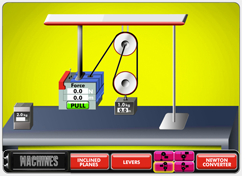# MachinesHow many people does it take to lift a 14,000 pound African elephant? One if you have the correct machine. And that’s no joke. Machines come in various types and are used for many purposes. You probably use hundreds of machines during the course of your typical day. In this simulation, you’ll test three major types of machines to better understand their operation. Now you better get started because you have lots of work to do.

### What is force?

A force can simply be defined as any push or pull. However, a more in-depth definition can be applied to the phrase "net force." A net force is any push or pull that causes a change in the motion or shape of an object. If a car is standing still, a net force is needed to start the car moving. If the car's moving, a net force is required to slow it down, speed it up or to change its direction.

### What is a newton?

In the metric system, force is measured in a unit called the newton, abbreviated as "N." It's very important that you avoid confusing the terms mass, measured in grams or kilograms, with force. Mass is not a force, so therefore, grams or kilograms are never used as the units to measure force.

### What is distance?

For a lever, distance is typically measured from the point where a force is applied to the fulcrum. The input distance would be the length from the fulcrum to the applied or input force. The output distance is the length from the fulcrum to the load or output force. A pulley measures the input distance as the length moved by the applied force, the output distance is the length the load is lifted.

### What is work?

The physics definition of work is force times the distance an object is moved by the force. The metric unit of energy is called the joule, abbreviated as "J." One joule is defined as 1 newton times 1 meter. The definition of work implies that an object must be moved for work to be done on the object. A person holding a heavy box above her head takes energy, but the person does no work to support the box while positioned above her head.

### What is efficiency?

Efficiency is a measure of the work out of a machine versus the work put into the machine. The higher the efficiency of a machine, the more effective the machine is in completing work. The equation for efficiency is: efficiency = (work out/work in) × 100%. Since all machines have moving parts that experience friction, no machine can be 100% efficient because some of the input energy is converted to waste heat that does not result in useful output work done by the machine.

### What is an inclined plane?

An inclined plane is a simple machine in the form of a ramp. The height of the ramp and the distance covered by the ramp determine the mechanical advantage of the ramp. A very steep ramp would have a low mechanical advantage and the force required to move a heavy object up the ramp would be large. A long, flatter ramp with a higher mechanical advantage would take much less force to move the same object up to a similar height; however, the distance the object was moved along the ramp would be greatly increased.

### What is a lever?

A lever is a simple machine consisting of a rigid bar and a supporting fulcrum. The mechanical advantage of a lever varies depending on the position of the applied force, load, and fulcrum. The positioning of these three lever components defines the class of the lever as being 1st, 2nd, or 3rd class. Levers are generally used to reduce the required input force or increase the speed of the load.

### What is a pulley?

A pulley is a simple machine consisting of a wheel and axle with a rope or string looped over the wheel. Pulley systems can be comprised of single or multiple pulleys, and the pulleys can be fixed or moveable. Pulleys are used to change the direction of the applied force or to reduce the required applied force to lift a heavier load. Like all machines, any reduction in the applied force must be accompanied by an increase in the input distance moved to ensure that work out never is greater than work in.